Gel'fond-Baker method

(Redirected from Gel'fond–Baker method)

for Diophantine equations

One of the most efficient methods for obtaining explicit upper bounds on the size of integer solutions of some broad classes of Diophantine equations arises from the theory of transcendental numbers, from the solution by A.O. Gel'fond of Hilbert's seventh problem and from subsequent work of A. Baker. The Gel'fond–Baker method also incorporates ideas due to C.L. Siegel, K. Mahler and S. Lang. From this method, explicit upper bounds have been derived for:

1) integer points on curves of genusand(effective versions of Siegel's theorem, due to Baker, J. Coates and W.M. Schmidt); [a2], Chapt. IV, Sect. 12;

2) Thue equations, whereis a homogeneous polynomial with algebraic coefficients,is a fixed non-zero algebraic number, and the polynomial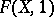has at least three distinct roots; [a1], Chapt. 5; [a2], Chapt. 3; [a3], Chapt. 4–5;

3) hyper-elliptic and super-elliptic equations, namely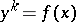whereis a fixed integer andis a polynomial with algebraic coefficients, which define curves of positive genus. This is the case whenandhas at least three distinct roots or when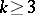andhas at least two distinct roots; [a2], Chapt. 4; [a3], Chapt. 6. The method also applies to some classes of norm-form equations in more than two variables (by the work of K. Győry).

The bounds obtained from this method are typically extremely large, and some further results on Diophantine approximations are required to be able to completely determine all the solutions. Upper estimates are obtained for the height of the solutions, either in the ring of integers of a fixed algebraic number field, or, more generally, in the ring of-integers, whereis a fixed finite set of prime ideals of(cf. also Ideal; Prime ideal). This means that a denominator forandis allowed, but is required to be the product of primes from a fixed finite set. This generalizes ordinary integers and units, which arise from taking forthe empty set. For instance, for the field of rational numbers, given a finite set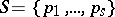of prime numbers, the-integers are the rational numbers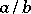such that all prime divisors of the denominatorbelong to, while the-units are the rational numbers of the form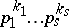with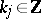(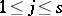).

Furthermore, the method can be extended in some cases to allow the exponents onandto vary. The most celebrated example is Tijdeman's theorem on the Catalan equation [a1], Chapt. 12, [a3], Chapt. 7, Sect. 3: There are only finitely many tuples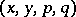of integers, all of them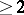, satisfying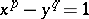.

According to Pillai's conjecture [a1], p. 201, [a2], p. 207, for each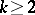the same should hold for the equation.

To demonstrate Baker's method, consider the Thue equation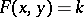, whereis a fixed non-zero algebraic number, whileis a homogeneous polynomial with algebraic coefficients such thathas at least three distinct roots,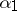,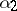,. The unknownsandare algebraic integers in a number field, which is assumed to contain not only the number, but also the coefficients ofas well as the roots of. Then one may write, for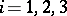,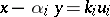, where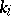belongs to a fixed finite subset of(independent of the solution), while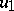,,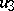are (unknown) units in. Eliminatingandfrom these three equations yields the relation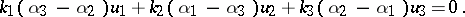Therefore, a key step in the Gel'fond–Baker method is the following result, which deals with the-unit equation: Ifis a number field andis a finite set of prime ideals of, the equation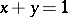has only finitely many solutions in-units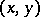of. Moreover, explicit bounds for the size of the solutions can be given.

The proof of this result relies on an estimate from transcendental number theory. Writeandusing a basisof the group of-units modulo torsion. Then a "large" solutionto the Diophantine equationgives rise to a "small" value for a number of the form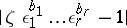, where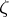is a root of unity andare rational integers. The so-called "theory of linear forms in logarithms" (cf. Linear form in logarithms) provides a lower bound for such numbers, which is sharp in terms of. This lower bound, together with the link between large solutions and small values, then provides an upper bound for the size of solutions.

The Schmidt subspace theorem and its-adic variants by H.P. Schlickewei [a2], Chapt.5, Sect. 1, imply more generally that for each, the Diophantine equation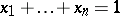has only finitely many solutions in-units of(one considers only solutions where no subsum on the left-hand side vanishes). But forthe result is not effective: upper bounds for the number of solutions can be given, but no bound for the size of the solutions is known in general.